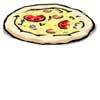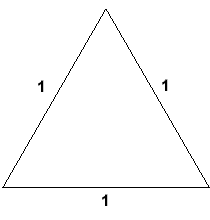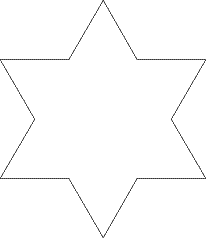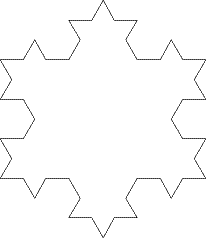#### You may also like### Pizza Portions

My friends and I love pizza. Can you help us share these pizzas equally?### Tweedle Dum and Tweedle Dee

Two brothers were left some money, amounting to an exact number of pounds, to divide between them. DEE undertook the division. "But your heap is larger than mine!" cried DUM...### Sum Equals Product

The sum of the numbers 4 and 1 [1/3] is the same as the product of 4 and 1 [1/3]; that is to say 4 + 1 [1/3] = 4 � 1 [1/3]. What other numbers have the sum equal to the product and can this be so for any whole numbers?

# Smaller and Smaller

##### Age 7 to 14 Challenge Level:

Here is an equilateral triangle which has sides of length 1 unit:We could take out the middle third of each side and replace it with a spike. If the spike is made of two lines which are the same length as the missing section, it will look like this:We could do the same again. Taking out the middle third of each line and replacing it with a spike in the same way gives this:Remembering that the length of each side of our original equilateral triangle is 1 unit, what is the perimeter of the first shape we drew above? And the second? Can you see a pattern? Perhaps you can explain why this pattern comes about.

Can you predict, without drawing, what the perimeter of the next shape will be if we continue drawing them in the same way?

Could you predict the perimeter of any shape in the series?

The shapes above are called fractals. A fractal is made up of lots of copies of itself, each one smaller than the last. See also the problems Von Koch Curve, The Cantor Set and How Long is The Cantor Set?